# Eta-invariant-invariant

Letbe an unbounded self-adjoint operator with only pure point spectrum (cf. also Spectrum of an operator). Letbe the eigenvalues of, counted with multiplicity. Ifis a first-order elliptic differential operator on a compact manifold, then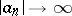and the seriesis convergent for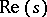large enough. Moreover,has a meromorphic continuation to the complex plane, witha regular value (cf. also Analytic continuation). The value ofatis called the eta-invariant of, and was introduced by M.F. Atiyah, V.K. Patodi and I.M. Singer in the foundational paper [a1] as a correction term for an index theorem on manifolds with boundary (cf. also Index formulas). For example, in that paper, they prove that the signature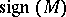of a compact, oriented,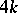-dimensional Riemannian manifold with boundarywhose metric is a product metric near the boundary is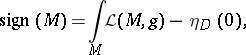where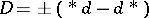is the signature operator on the boundary and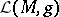the Hirzebruch-polynomial associated to the Riemannian metric on.

The definition of the eta-invariant was generalized by J.-M. Bismut and J. Cheeger in [a2], where they introduced the eta-form of a family of elliptic operators as above. It can be used to recover the eta-invariant of operators in the family.